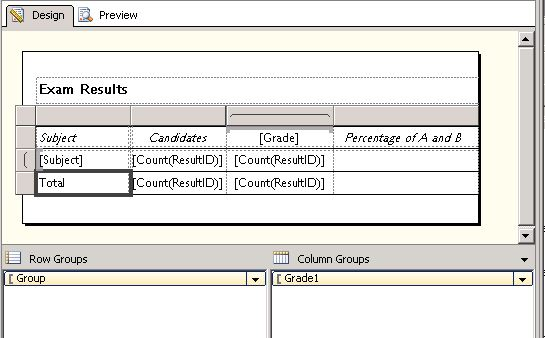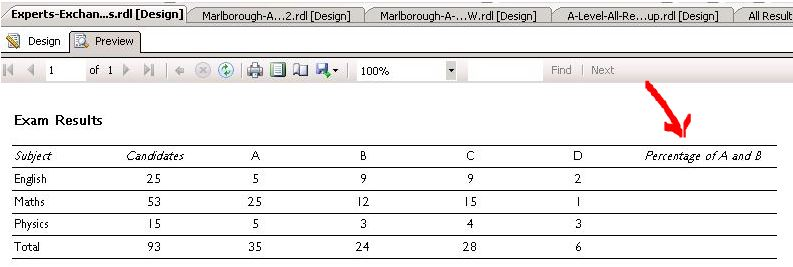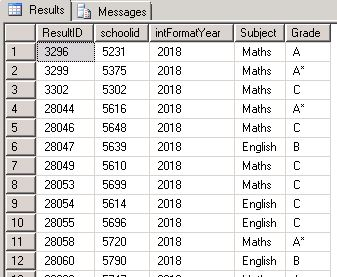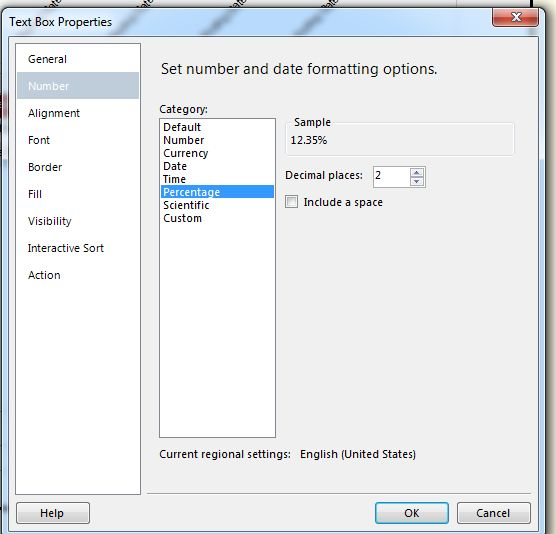An SSRS expression to calculate percentagesict support used Ask the Experts™
on
Hello,

I am preparing exam results reports in SSRS using Visual Studio 2008, and the actual report is visually very simple.

I have attached a simplified version here, and it simply lists subjects, grades, and the total of each grade.

I am trying to add a column at the end, to show the percentage of both A and B grades together, within all grades (A,B,C,D).

So, for example, in English, out of a total of 25 candidates, 14 had A or B, so the percentage of A,B is 56%

I'm struggling to write an expression to do this. I would normally do a expression, consisting of a calculation, and reference textboxes in the report grid
to get the values, but in this case the data is grouped, so I'm not sure what to do.

I can't find any similar example on the web.

I have attached sample of the report preview, rendered report, and the SQL output grid showing all the field names.

Can anyone help, please?

Many thanks,

Jim DoransComment
Watch Question

Do more withEXPERT OFFICE® is a registered trademark of EXPERTS EXCHANGE®
Database Developer
Top Expert 2014

Commented:
That would be tricky, given your column matrix. It should be easier to do this in your SQL query: if you share that, I can work something up for you.

Commented:
* Rename the Candidates textbox to Candidates
* Add a percentage-format to the textbox holding the below expression (note: I converted the denominator to Decimal because I think SSRS does that weird thing where int / int = int):
=SUM( IIF(Fields!Grade.Value = "A" or Fields!Grade.Value = "B", 1, 0) ) / CDec( ReportItems!Candidates.Value )

Commented:
John Vidmar :

Many thanks for your help here.

Your proposed solution, in principle, worked perfectly. It did return a number in the format nn.nnnnnn , so I amended it as follows :

old :
=SUM( IIF(Fields!Grade.Value = "A" or Fields!Grade.Value = "B", 1, 0) ) / CDec( ReportItems!Candidates.Value )
new :
= ROUND( SUM ( IIF(Fields!Grade.Value = "A" or Fields!Grade.Value = "B", 1, 0) ) / ( ReportItems!Candidates.Value ) * 100,0)
...in order to round to whole numbers.

Now, in the totals row, I want to add an expression in the bottom right box, to show the average of all the percentages. I encapsulated the above ROUND statement in a AVG() function, but got the error "aggregate functions can only be used on report items contained in page headers and footers".

Presumably this is because it is trying to average amounts that have not yet been calculated?

Do you think there is a solution for this please?

Thanks again!

Jim

Commented:
Russell Fox : I have chosen Jon Vidmar's SSRS solution, but I would very much like the option of doing this in SQL. My successor will be advanced in SQL, but not so experienced in SSRS.

As there will be many more exams reports to write, an SQL solution would be most useful.

The SSRS code is this :
SELECT * FROM  Grades WHERE Grade IN ('A', 'B', 'C', 'D')

This is the sample code to create and populate table (full code script is attached in a file) :

[ResultID] [int] NOT NULL,
[schoolid] [nvarchar](4) NULL,
[intFormatYear] [int] NULL,
[Subject] [nvarchar](255) NULL,
)

INSERT [dbo].[Grades] ([ResultID], [schoolid], [intFormatYear], [Subject], [Grade]) VALUES (3296, N'5231', 2018, N'Maths', N'A')
INSERT [dbo].[Grades] ([ResultID], [schoolid], [intFormatYear], [Subject], [Grade]) VALUES (3299, N'5375', 2018, N'Maths', N'A

INSERT [dbo].[Grades] ([ResultID], [schoolid], [intFormatYear], [Subject], [Grade]) VALUES (28726, N'5668', 2018, N'Physics', N'A*')

Many thanks!

Jim
ee-create-populate-table.sql
Database Developer
Top Expert 2014

Commented:
Gotcha, I'd use a CTE:

DECLARE @Grades TABLE (
[ResultID] [int] NOT NULL,
[schoolid] [nvarchar](4) NULL,
[intFormatYear] [int] NULL,
[Subject] [nvarchar](255) NULL,
)

(
[ResultID]
, [schoolid]
, [intFormatYear]
, [Subject]
)
VALUES (3296, N'5231', 2018, N'Maths', N'A')
,(3299, N'5375', 2018, N'Maths', N'A*')
,(3302, N'5302', 2018, N'Maths', N'C')
,(28044, N'5616', 2018, N'Maths', N'A*')
,(28046, N'5648', 2018, N'Maths', N'C')
,(28047, N'5639', 2018, N'English', N'B')
,(28049, N'5610', 2018, N'Maths', N'C')
,(28053, N'5699', 2018, N'Maths', N'C')
,(28054, N'5614', 2018, N'English', N'C')
,(28055, N'5696', 2018, N'English', N'C')
,(28058, N'5720', 2018, N'Maths', N'A*')
,(28060, N'5790', 2018, N'English', N'B')
,(28063, N'5747', 2018, N'Maths', N'A')
,(28065, N'5739', 2018, N'Maths', N'A*')
,(28067, N'5773', 2018, N'English', N'A')
,(28069, N'5730', 2018, N'Maths', N'A')
,(28071, N'5727', 2018, N'Maths', N'A')
,(28073, N'5727', 2018, N'Maths', N'A')
,(28075, N'5769', 2018, N'Maths', N'C')
,(28076, N'5707', 2018, N'English', N'C')
,(28078, N'5771', 2018, N'Maths', N'C')
,(28080, N'5716', 2018, N'Maths', N'C')
,(28081, N'5750', 2018, N'English', N'B')
,(28083, N'5713', 2018, N'Maths', N'A*')
,(28086, N'5809', 2018, N'Maths', N'B')
,(28089, N'5805', 2018, N'Maths', N'B')
,(28090, N'5851', 2018, N'English', N'D')
,(28092, N'5836', 2018, N'Maths', N'C')
,(28094, N'5823', 2018, N'Maths', N'B')
,(28096, N'5848', 2018, N'Maths', N'C')
,(28098, N'5848', 2018, N'Maths', N'A*')
,(28100, N'5917', 2018, N'Maths', N'A')
,(28102, N'5973', 2018, N'Maths', N'B')
,(28104, N'5947', 2018, N'Maths', N'C')
,(28106, N'5915', 2018, N'Maths', N'C')
,(28108, N'5973', 2018, N'Maths', N'A')
,(28109, N'5967', 2018, N'English', N'B')
,(28111, N'5996', 2018, N'Maths', N'B')
,(28112, N'5922', 2018, N'English', N'A')
,(28114, N'5971', 2018, N'Maths', N'A')
,(28115, N'5992', 2018, N'English', N'C')
,(28117, N'5927', 2018, N'Maths', N'C')
,(28119, N'5994', 2018, N'Maths', N'C')
,(28121, N'5931', 2018, N'Maths', N'A*')
,(28123, N'5919', 2018, N'English', N'B')
,(28125, N'0015', 2018, N'Maths', N'A*')
,(28127, N'0024', 2018, N'Maths', N'A')
,(28129, N'0036', 2018, N'English', N'A')
,(28132, N'0022', 2018, N'Maths', N'A*')
,(28134, N'0057', 2018, N'Maths', N'A')
,(28136, N'0037', 2018, N'Maths', N'A*')
,(28139, N'0067', 2018, N'Maths', N'B')
,(28141, N'0021', 2018, N'Maths', N'B')
,(28142, N'0024', 2018, N'English', N'C')
,(28144, N'0084', 2018, N'Maths', N'A')
,(28146, N'0029', 2018, N'Maths', N'E')
,(28148, N'0071', 2018, N'Maths', N'A')
,(28150, N'0109', 2018, N'Maths', N'A*')
,(28152, N'0100', 2018, N'Maths', N'B')
,(28154, N'0164', 2018, N'Maths', N'A')
,(28155, N'0161', 2018, N'English', N'B')
,(28157, N'0191', 2018, N'Maths', N'A')
,(28158, N'0125', 2018, N'English', N'C')
,(28160, N'0187', 2018, N'Maths', N'A*')
,(28164, N'0163', 2018, N'Maths', N'A')
,(28165, N'0119', 2018, N'English', N'D')
,(28167, N'0119', 2018, N'Maths', N'B')
,(28168, N'0190', 2018, N'English', N'C')
,(28169, N'0183', 2018, N'English', N'A*')
,(28171, N'0259', 2018, N'Maths', N'A')
,(28173, N'0258', 2018, N'Maths', N'A*')
,(28175, N'0205', 2018, N'English', N'C')
,(28177, N'0295', 2018, N'Maths', N'A')
,(28179, N'0200', 2018, N'Maths', N'A')
,(28180, N'5863', 2018, N'English', N'C')
,(28182, N'0159', 2018, N'Maths', N'B')
,(28184, N'1457', 2018, N'Maths', N'A*')
,(28187, N'5978', 2018, N'Maths', N'C')
,(28189, N'1467', 2018, N'Maths', N'D')
,(28190, N'1477', 2018, N'English', N'B')
,(28192, N'1147', 2018, N'Maths', N'A')
,(28194, N'1775', 2018, N'Maths', N'A')
,(28195, N'1775', 2018, N'English', N'A*')
,(28197, N'1527', 2018, N'Maths', N'B')
,(28198, N'1427', 2018, N'English', N'B')
,(28200, N'1367', 2018, N'Maths', N'C')
,(28202, N'1567', 2018, N'Maths', N'E')
,(28203, N'1637', 2018, N'English', N'A')
,(28206, N'1697', 2018, N'Maths', N'A')
,(28208, N'1647', 2018, N'Maths', N'A*')
,(28210, N'1417', 2018, N'Maths', N'A')
,(28212, N'1677', 2018, N'Maths', N'A')
,(28214, N'1687', 2018, N'Maths', N'A*')
,(28216, N'1377', 2018, N'Maths', N'A*')
,(28219, N'1467', 2018, N'Maths', N'A*')
,(28221, N'1467', 2018, N'English', N'A')
,(28223, N'1357', 2018, N'Maths', N'A')
,(28225, N'1657', 2018, N'Maths', N'A*')
,(28227, N'5668', 2018, N'Maths', N'A*')
,(28229, N'1617', 2018, N'English', N'B')
,(28232, N'1348', 2018, N'Maths', N'B')
,(28234, N'1436', 2018, N'Maths', N'A')
,(28236, N'1492', 2018, N'Maths', N'A*')
,(28238, N'1281', 2018, N'Maths', N'A*')
,(28536, N'5648', 2018, N'Physics', N'D')
,(28547, N'5699', 2018, N'Physics', N'B')
,(28572, N'5727', 2018, N'Physics', N'A*')
,(28580, N'5716', 2018, N'Physics', N'D')
,(28584, N'5809', 2018, N'Physics', N'C')
,(28587, N'5836', 2018, N'Physics', N'D')
,(28634, N'0022', 2018, N'Physics', N'A')
,(28637, N'0037', 2018, N'Physics', N'A*')
,(28646, N'0084', 2018, N'Physics', N'B')
,(28657, N'0100', 2018, N'Physics', N'C')
,(28658, N'0164', 2018, N'Physics', N'A')
,(28661, N'0187', 2018, N'Physics', N'A*')
,(28673, N'0258', 2018, N'Physics', N'A')
,(28686, N'1457', 2018, N'Physics', N'A')
,(28689, N'5978', 2018, N'Physics', N'C')
,(28692, N'1467', 2018, N'Physics', N'C')
,(28696, N'1775', 2018, N'Physics', N'B')
,(28719, N'1377', 2018, N'Physics', N'A*')
,(28722, N'1467', 2018, N'Physics', N'A')
,(28726, N'5668', 2018, N'Physics', N'A*')

; WITH [ABTotal]
AS (SELECT [Subject], ABTotal = COUNT(1)
WHERE [Grade] = 'A' -- WHERE LEFT([Grade], 1) = 'A' --(if A* should be included)
OR [Grade] = 'B'
GROUP BY [Subject]
)
SELECT [g].[ResultID]
, [g].[schoolid]
, [g].[intFormatYear]
, [g].[Subject]
, [a].[ABTotal]
LEFT JOIN [ABTotal] a
ON [a].[Subject] = [g].[Subject]

Commented:
Russell Fox + John Vidmar :

Thank you both for your different but valuable solutions.

Jim

Commented:
Hello, I closed this prematurely - I should have waited for an answer to the second part of my question :

Now, in the totals row, I want to add an expression in the bottom right box, to show the average of all the percentages. I encapsulated the above ROUND statement in a AVG() function, but got the error "aggregate functions can only be used on report items contained in page headers and footers".

Presumably this is because it is trying to average amounts that have not yet been calculated?

Do you think there is a solution for this please? Sorry for the confusion.

Thanks again!

Commented:
I originally mentioned adding a textbox-format:  Add a percentage-format to the textbox holding the below expression

With the proper format (I think 0%), you don't need round-function, or multiplying by 100.

Please show a picture of the expression location in your matrix.

Commented:
Hi John,

Thank you for your continuing support here.

The attached pic shows your proposed expression, and my amended version of that.

This is what is looks like, based on my understanding of your answer.

The top section shows the matrix - the red arrow point shows the textbox which I renamed to "Candidates".

The blue arrow point shows the box where your expression was entered :

=SUM( IIF(Fields!Grade.Value = "A" or Fields!Grade.Value = "B", 1, 0) ) / CDec( ReportItems!Candidates.Value )

… and the middle section of the pic shows the result, with lots of decimal places.

The bottom section of the pic shows the result when I used the amended expression :

= ROUND( SUM ( IIF(Fields!Grade.Value = "A" or Fields!Grade.Value = "B", 1, 0) ) / ( ReportItems!Candidates.Value ) * 100,0)

… and as you can see, it shows whole numbers, and the percentages are correct.

The green arrow point shows where I am trying to create an expression which will give the average of the percentages, in other words, the row percentages are 56, 70, 53 ...so the average would be 60.

This is now what I am trying to achieve.

I attempted this : I encapsulated the above ROUND statement in a AVG() function, but got the error "aggregate functions can only be used on report items contained in page headers and footers".

Presumably this is because it is trying to average amounts that have not yet been calculated?

This is what I am struggling with now.

I hope I have presented everything clearly, so you can spot if I have done something wrong, or misunderstood your original advice.

Thanks.
ee-combined.jpg
Commented:
Alter the expression pointed by the green-arrow to use a simple count-aggregate-function in the denominator instead of referencing a TextBox value (ReportItems):
=SUM( IIF(Fields!Grade.Value = "A" or Fields!Grade.Value = "B", 1, 0) ) / CDec( Count(Fields!ResultID.Value) )
This is the graphical technique to add a Percentage-Format, this resulting-format can then be viewed/altered on the properties-pane, which you can copy/paste to other percentage-textboxes, the below example will display a value of 0.12345 as 12.35% (note the last digit rounding):Commented:
@John_Vidmar - thank you for your solution. it works just fine.

Jim

Do more withSubmit tech questions to Ask the Experts™ at any time to receive solutions, advice, and new ideas from leading industry professionals.